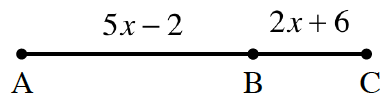### Home > GC > Chapter 1 > Lesson 1.2.1 > Problem1-56

1-56.

The distance along a straight road is measured as shown in the diagram below. If the distance between towns $\text{A}$ and $\text{C}$ is $67$ miles, find the distance between towns $\text{A}$ and $\text{B}$.$5x-2+2x+6=67$